# 问题背景

• 信息选择：聚焦式自上而下地选择重要信息，过滤掉无关的信息，也就是注意力机制。
• 外部记忆： 优化神经网络的记忆结构，使用额外的外部记忆，来提高网络的存储信息的容量，即记忆力机制。

• 聚焦式注意力：自上而下有意识的注意力。有预定目的、依赖任务、主动有意识的聚焦于某一对象的注意力。
• 显著性注意力：自下而上无意识的注意力。由外界刺激驱动的注意力，无需主动干预，也和任务无关。如Max Pooling和Gating。

# 普通注意力机制

## 加性注意力

### LSTM计算过程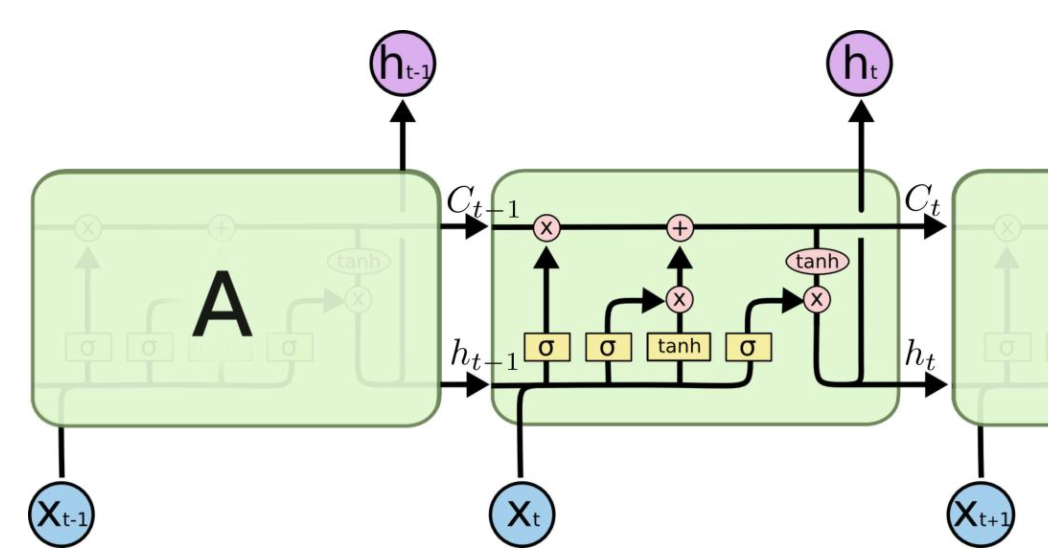• 计算在该时刻的预测值：$\hat{y}_{t}=\sigma\left(h_{t} * w+b\right)$
• 计算下个时间片的隐节点状态：$h_t$

LSTM在计算h_t时增加门控机制：

• $f_{t}=\sigma\left(W_{f} \cdot\left[h_{t-1}, x_{t}\right]+b_{f}\right)$
• $i_{t}=\sigma\left(W_{i} \cdot\left[h_{t-1}, x_{t}\right]+b_{i}\right)$
• $\tilde{C}_{t}=\tanh \left(W_{C} \cdot\left[h_{t-1}, x_{t}\right]+b_{C}\right)$
• $h_{t}=o_{t} * \tanh \left(C_{t}\right)$

### GRU计算过程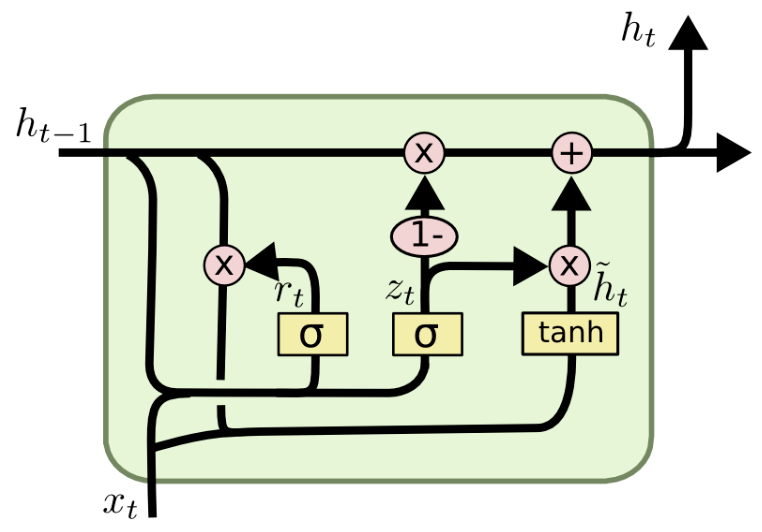• $z_{t}=\sigma\left(W_{z} \cdot\left[h_{t-1}, x_{t}\right]\right)$
• $r_{t}=\sigma\left(W_{r} \cdot\left[h_{t-1}, x_{t}\right]\right)$
• $\tilde{h}_{t}=\tanh \left(W \cdot\left[r_{t} * h_{t-1}, x_{t}\right]\right)$
• $h_{t}=\left(1-z_{t}\right) h_{t-1}+z_{t} \tilde{h}_{t}$

### Encoder-Decoder计算过程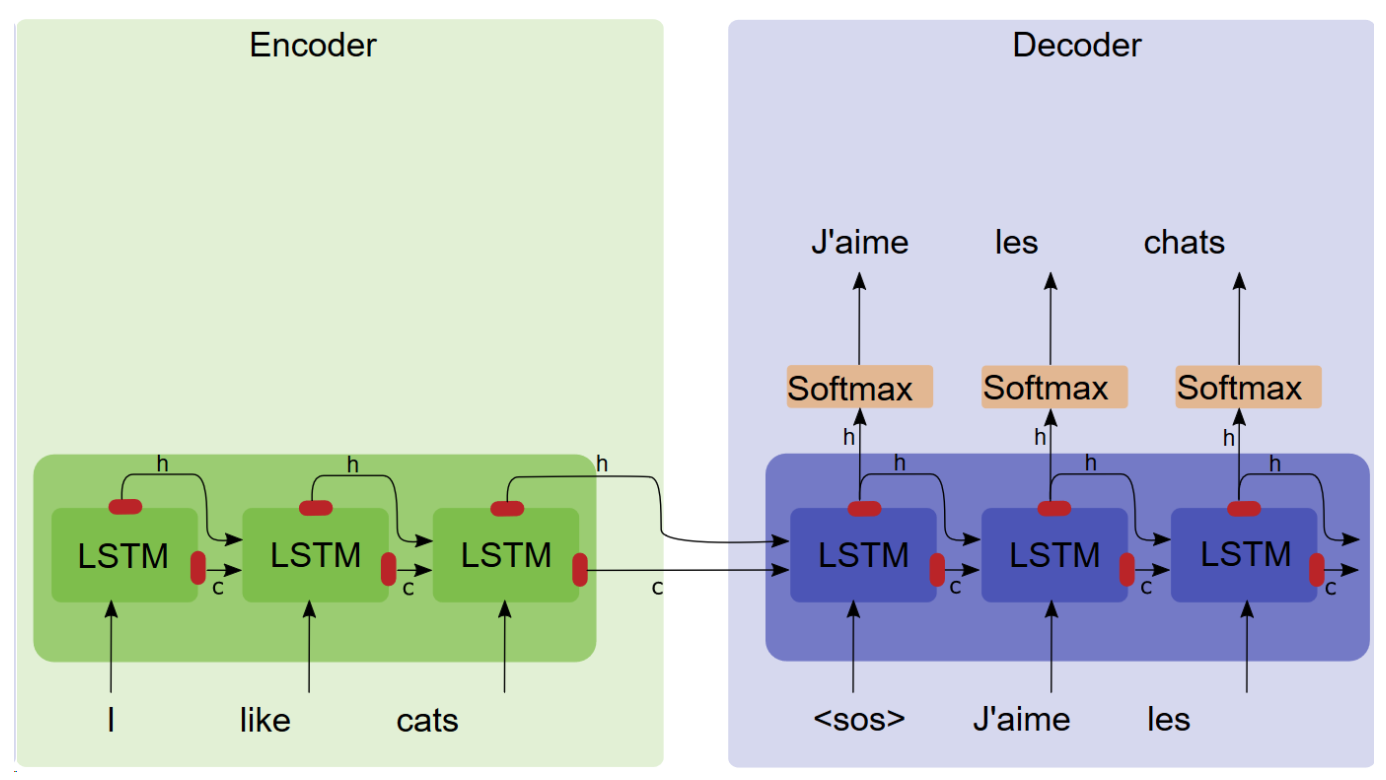### Encoder-Decoder with Bahdanau Attention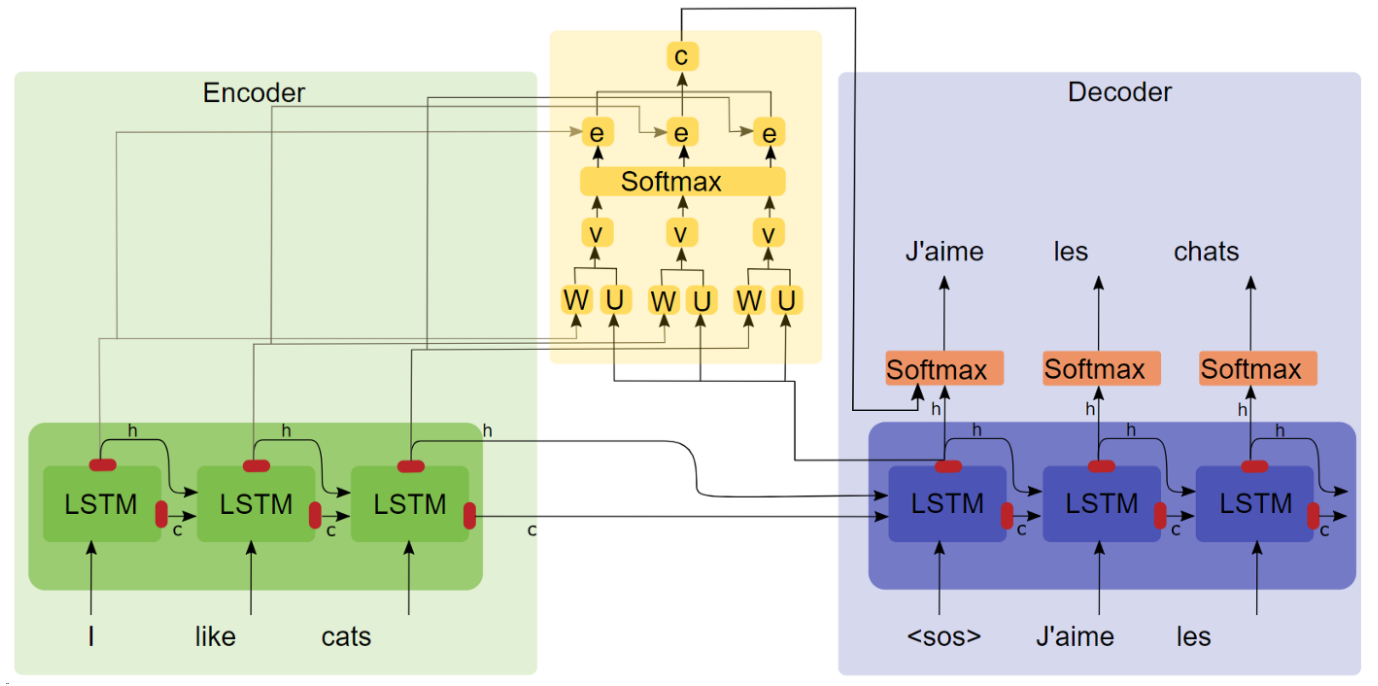• Encoder
• $h_{i}=\tanh \left(W\left[h_{i-1}, x_{i}\right]\right)$
• $o_{i}=\operatorname{softmax}\left(V h_{i}\right)$
• Decoder
• 生成该时刻的语义向量
• $e_{t i}=v_{a}^{\top} \tanh \left(W_{a}\left[s_{i-1}, h_{i}\right]\right)$：Encoder中第 i 时刻 Encoder隐层状态 $h_i$ 对Decoder中 $t$ 时刻隐层状态 $s_t$ 的影响程度
• $\alpha_{t i}=\frac{\exp \left(e_{t i}\right)}{\sum_{k=1}^{T} \exp \left(e_{t k}\right)}$：对$e_{ti}$的softmax归一化
• $c_{t}=\sum_{i=1}^{T} \alpha_{t i} h_{i}$
• 传递隐层信息并预测
• $s_{t}=\tanh \left(W\left[s_{t-1}, y_{t-1}, c_{t}\right]\right)$
• $o_{t}=\operatorname{softmax}\left(V s_{t}\right)$

## 双线性注意力

• Encoder：和Bahdanau Attention一样
• Decoder
• 生成该时刻的语义向量
• $s_{t}=\tanh \left(W\left[s_{t-1}, y_{t-1}\right]\right)$
• $e_{t i}=s_{t}^{\top} W_{a} h_{i}$
• $\alpha_{t i}=\frac{\exp \left(e_{t i}\right)}{\sum_{k=1}^{T} \exp \left(e_{t k}\right)}$
• $c_{t}=\sum_{i=1}^{T} \alpha_{t i} h_{i}$
• 传递隐层信息并预测
• $\tilde{s}_{t}=\tanh \left(W_{c}\left[s_{t}, c_{t}\right]\right)$
• $o_{t}=\operatorname{softmax}\left(V \tilde{s}_{t}\right)$

## 点击注意力• 信息输入：用X = [x1, · · · , xN ]表示N 个输入信息
• 注意力分布计算：令Key=Value=X，则可以给出注意力分布$\alpha_{i}=\operatorname{softmax}\left(s\left(k e y_{i}, q\right)\right)=\operatorname{softmax}\left(s\left(X_{i}, q\right)\right)$
• 我们将$\alpha_{i}$称之为注意力分布，$s\left(X_{i}, q\right)$为注意力打分机制，有几种打分机制
• 加性模型：$s\left(\mathbf{x}_{i}, \mathbf{q}\right)=\mathbf{v}^{\mathrm{T}} \tanh \left(W \mathbf{x}_{i}+U \mathbf{q}\right)$
• 点击模型：$s\left(\mathbf{x}_{i}, \mathbf{q}\right)=\mathbf{x}_{i}^{\mathrm{T}} \mathbf{q}$
• 缩放点击模型：$s\left(\mathbf{x}_{i}, \mathbf{q}\right)=\frac{\mathbf{x}_{i}^{\mathrm{T}} \mathbf{q}}{\sqrt{d}}$
• 双线性模型：$s\left(\mathbf{x}_{i}, \mathbf{q}\right)=\mathbf{x}_{i}^{\mathrm{T}} W \mathbf{q}$
• 信息加权平均：注意力分布$\alpha_{i}$可以解释为在上下文查询q时，第i个信息受关注的程度，采用一种“软性”的信息选择机制对输入信息X进行编码为：$\operatorname{att}(q, X)=\sum_{i=1}^{N} \alpha_{i} X_{i}$
• 这种编码方式为软性注意力机制（soft Attention），软性注意力机制有两种：普通模式（Key=Value=X）和键值对模式（Key!=Value），如下图中的左图和右图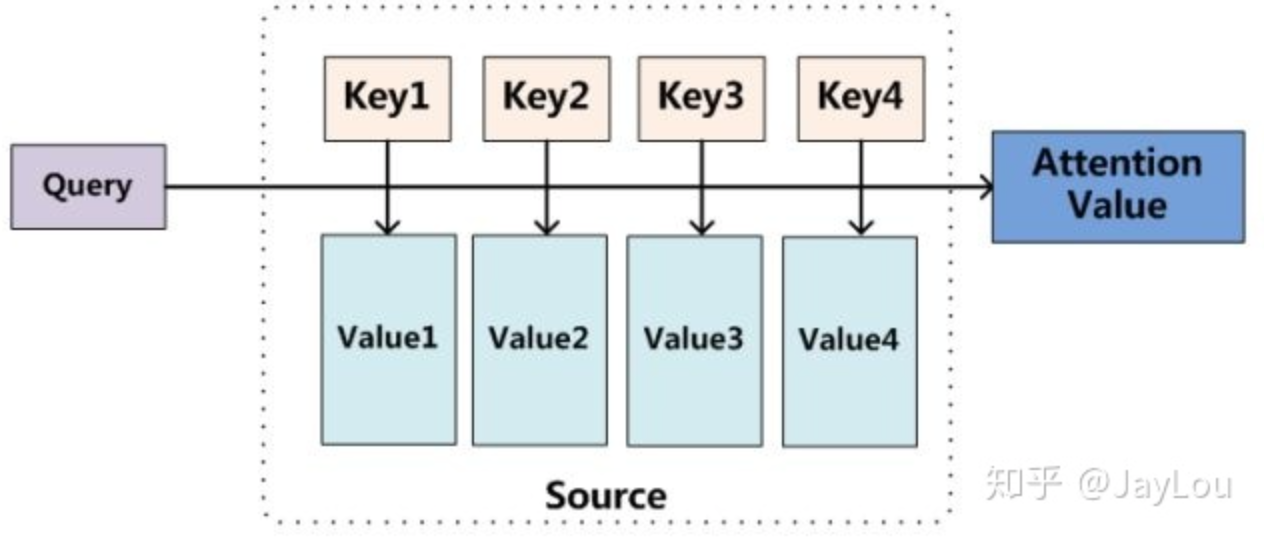# 注意力机制变体

Attention上的变种主要有3种：

• 硬性注意力：之前提到的注意力是软性注意力，其选择的信息是所有输入信息在注意力分布下的期望。还有一种注意力是只关注到某一个位置上的信息，叫做硬性注意力（hard attention）。硬性注意力有两种实现方式：
• 一种是选取最高概率的输入信息；
• 另一种硬性注意力可以通过在注意力分布式上随机采样的方式实现。
• 硬性注意力模型的缺点：
• 硬性注意力的一个缺点是基于最大采样或随机采样的方式来选择信息。因此最终的损失函数与注意力分布之间的函数关系不可导，因此无法使用在反向传播算法进行训练。为了使用反向传播算法，一般使用软性注意力来代替硬性注意力。硬性注意力需要通过强化学习来进行训练。
• 键值对注意力：即上面说的Key！=Value
• 多头注意力：多头注意力（multi-head attention）是利用多个查询Q = [q1, · · · , qM]，来平行地计算从输入信息中选取多个信息。每个注意力关注输入信息的不同部分，然后再进行拼接：$\operatorname{att}((K, V), Q)=\operatorname{att}\left((K, V), \mathbf{q}_{1}\right) \oplus \cdots \oplus \operatorname{att}\left((K, V), \mathbf{q}_{M}\right)$
• 自注意力：self-Attention中的Q是对自身（self）输入的变换，而在传统的Attention中，Q来自于外部，具体代码可参考：https://cloud.tencent.com/developer/article/1451523

## 自注意力

### Sentence Embedding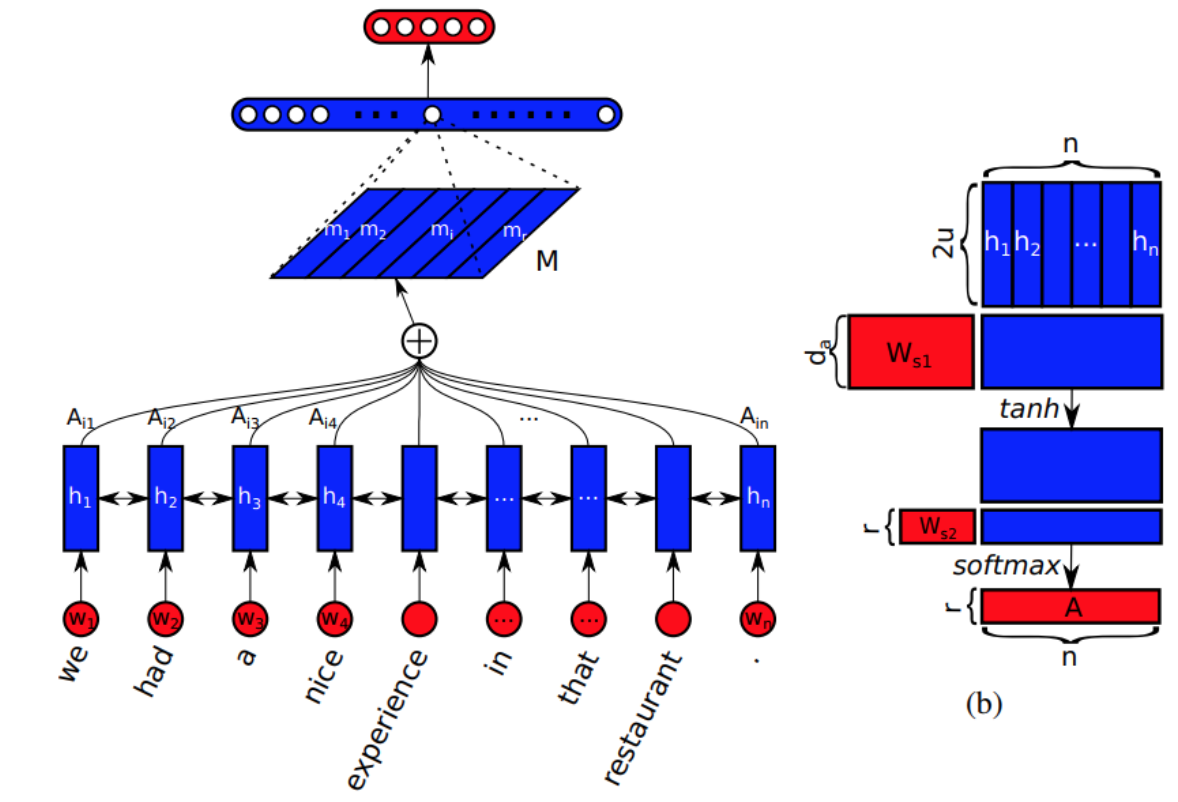• 给定一个句子，我们首先将其进行 Word embedding，得到：S = (w1, w2, … , wn)，然后讲这些 vector 拼成一个 2-D 的矩阵，维度为[n, d]
• 用双向 lstm 来建模，得到其两个方向的隐层状态，然后，此时我们可以得到维度为[n, 2*u]，记为H
• 为了将变长的句子，编码为固定长度的 embedding。论文通过选择 n 个 LSTM hidden states 的线性组合，来达到这一目标。计算这样的线性组合，需要利用 self-attention 机制，该机制将 lstm 的所有隐层状态 H 作为输入，并且输出为一个向量权重$\mathbf{a}=\operatorname{softmax}\left(\mathbf{w}_{\mathbf{s} \mathbf{2}} \tanh \left(W_{s 1} H^{T}\right)\right)$，其中W_{s1}维度是[d_a, 2*u]，w_{s2}是大小为d_a的向量
• 将 lstm 的隐层状态 H 和 attention weight a 进行加权，即可得到 attend 之后的向量 m
• 为了表示句子的总体的语义，需要多个 m 来聚焦于不同的部分。所以，需要用到multiple hops of attention，即想从句子中提取出 r 个不同的部分。论文将w_{s2}拓展为[r, d_a]的矩阵，记为W_{s2}，使得向量a变为矩阵A，即$A=\operatorname{softmax}\left(W_{s 2} \tanh \left(W_{s 1} H^{T}\right)\right)$，此时softmax是沿着输入的 第二个维度执行的，我们也可以把它看成是没有bias的2层的MLP。映射向量 m 然后就变成了[r, 2*u]的矩阵，我们通过将 annotation A 和 lstm 的隐层状态 H 进行相乘，得到的结果矩阵就是句子的映射M=AH

## 键值注意力

Transformer中使用呢了键值注意力，这里我们介绍一些其他论文中出现的key-value attention。

### Neural Language Modeling

• keys: 对比当前时刻的状态和过去时刻的状态
• values: 比较结果用于建模上下文
• prediction: 综合当前时刻和上下文的信息来进行预测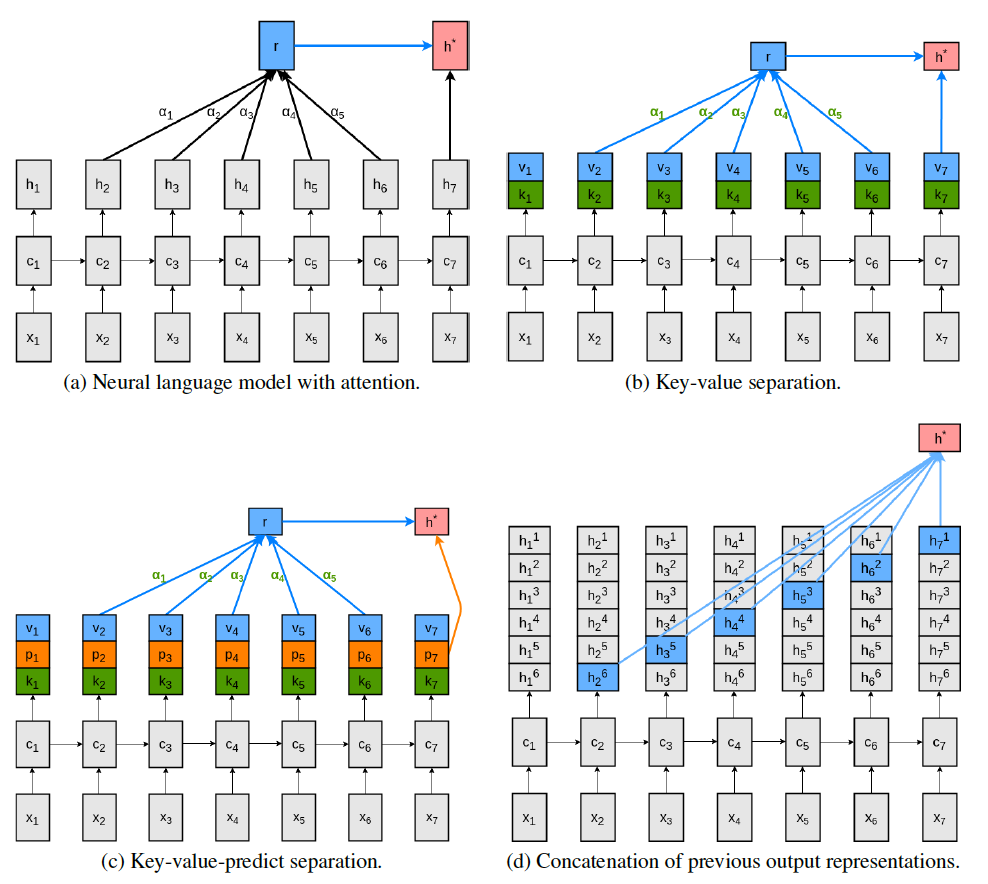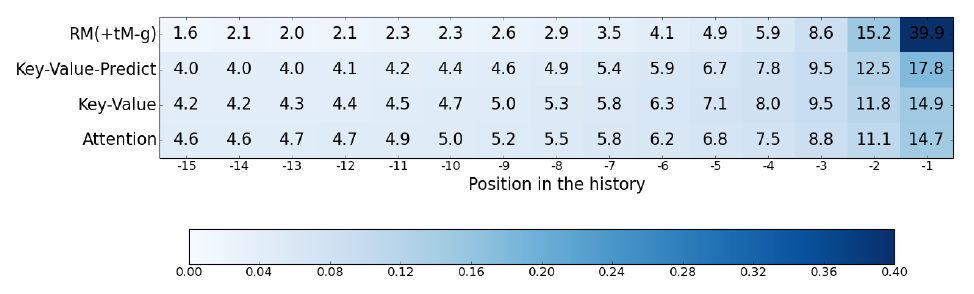## 相对位置自注意力

Transformer模型中注意力计算形式为$\text { attn }=\text { Attention }\left(X W^{Q}, X W^{K}, X W^{V}\right)$，其中表$X=X_{E}+X_{P}$示输入序列的WordEmbeddingPositionEncodings之和，在Attention函数中主要利用位置编码的地方在于计算AttnScore，而计算AttnScore的核心公式为：$\text { scores }=\left(X W^{Q}\right)\left(X W^{K}\right)^{T}=X W^{Q}\left(W^{K}\right)^{T} X^{T}$，经过变$W^{Q}\left(W^{K}\right)^{T}$换之后，位置编码信息会有所缺失。论文从结构上对AttentionMechanism进行改变，提出相对感知的注意力机制(Relationaware SelfAttention）。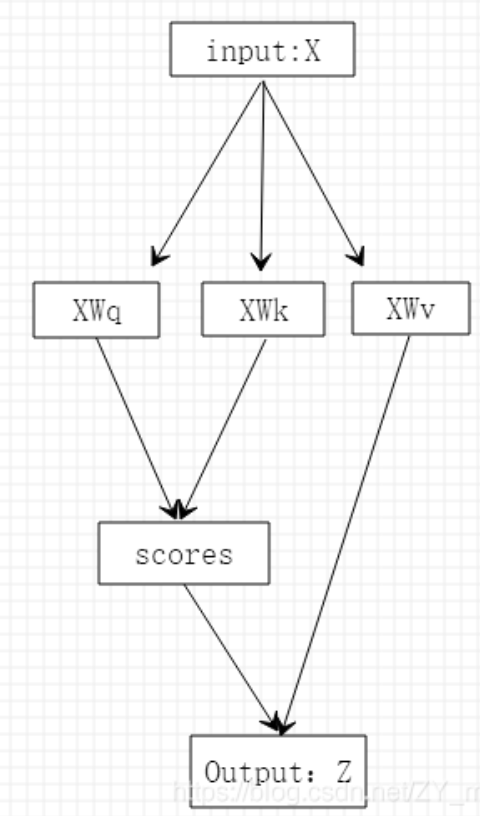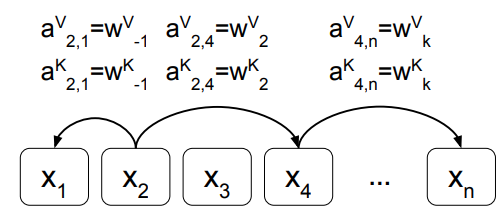\begin{aligned} a_{i j}^{K} &=w_{\mathrm{clip}(j-i, k)}^{K} \\ a_{i j}^{V} &=w_{\operatorname{clip}(j-i, k)}^{V} \\ \operatorname{clip}(x, k) &=\max (-k, \min (k, x)) \end{aligned}

$\operatorname{clip}(x, k)=\left\{\begin{array}{ll} -k & , x \leq-k \\ x & ,-k<x<k \\ k & , x \geq k \end{array}\right.$

## 层次注意力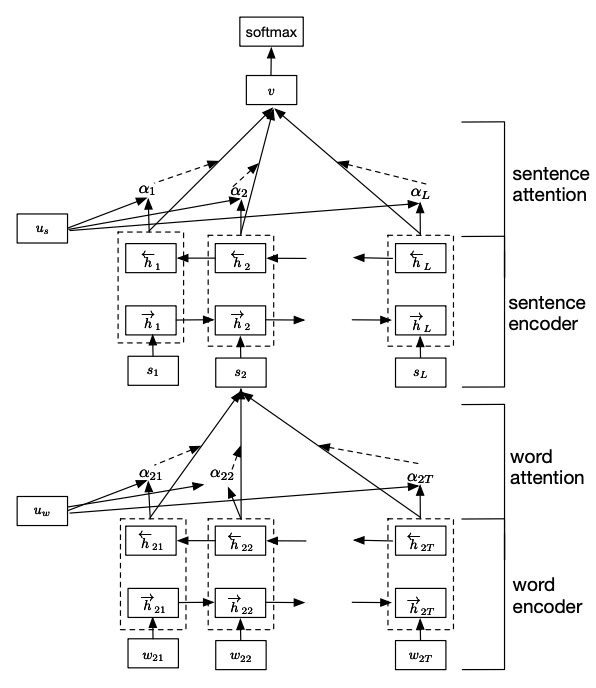• $x_{i t}=W_{e} w_{i t}, t \in[1, T]$
• $\vec{h}_{i t}=\overrightarrow{\operatorname{GRU}}\left(x_{i t}\right), t \in[1, T]$
• $\overleftarrow{h}_{i t}=\overleftarrow{\operatorname{GRU}}\left(x_{i t}\right), t \in[T, 1]$
• $h_{i t}=\left[\vec{h}_{i t}, \stackrel{\leftarrow}{h}_{i t}\right]$

• $r_{t}=\sigma\left(W_{r} x_{t}+U_{r} h_{t-1}+b_{r}\right)$，$r_t$是遗忘门，决定留下多少历史信息，如果$r_t$为0表示不保留历史信息
• $\tilde{h}_{t}=\tanh \left(W_{h} x_{t}+r_{t} \odot\left(U_{h} h_{t-1}\right)+b_{h}\right)$
• $z_{t}=\sigma\left(W_{z} x_{t}+U_{z} h_{t-1}+b_{z}\right)$，$z_t$控制历史信息和当前信息的保留比例
• $h_{t}=\left(1-z_{t}\right) \odot h_{t-1}+z_{t} \odot \tilde{h}_{t}$

• $u_{i t}=\tanh \left(W_{w} h_{i t}+b_{w}\right)$
• $\alpha_{i t}=\frac{\exp \left(u_{i t}^{\top} u_{w}\right)}{\sum_{t} \exp \left(u_{i t}^{\top} u_{w}\right)}$
• $s_{i}=\sum_{t} \alpha_{i t} h_{i t}$

• $\vec{h}_{i}=\overrightarrow{\operatorname{GRU}}\left(s_{i}\right), i \in[1, L]$
• $\overleftarrow{h}_{i}=\overleftarrow{\operatorname{GRU}}\left(s_{i}\right), t \in[L, 1]$
• $h_{i}=\left[\vec{h}_{i}, \overleftarrow{h}_{i}\right]$
• $u_{i}=\tanh \left(W_{s} h_{i}+b_{s}\right)$
• $\alpha_{i}=\frac{\exp \left(u_{i}^{\top} u_{s}\right)}{\sum_{i} \exp \left(u_{i}^{\top} u_{s}\right)}$
• $v=\sum_{i} \alpha_{i} h_{i}$

## 稀疏注意力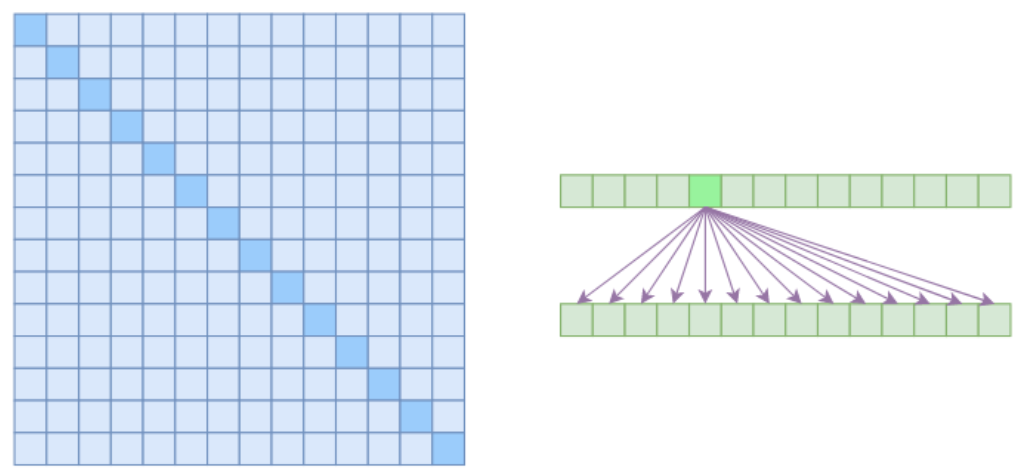### Atrous Self Attention

Atrous Self Attention的中文可以称之为“膨胀自注意力”、“空洞自注意力”、“带孔自注意力”等。Atrous Self Attention就是启发于“膨胀卷积（Atrous Convolution）”，如下右图所示，它对相关性进行了约束，强行要求每个元素只跟它相对距离为k,2k,3k,…的元素关联，其中k>1是预先设定的超参数。从下左的注意力矩阵看，就是强行要求相对距离不是k的倍数的注意力为0（白色代表0）：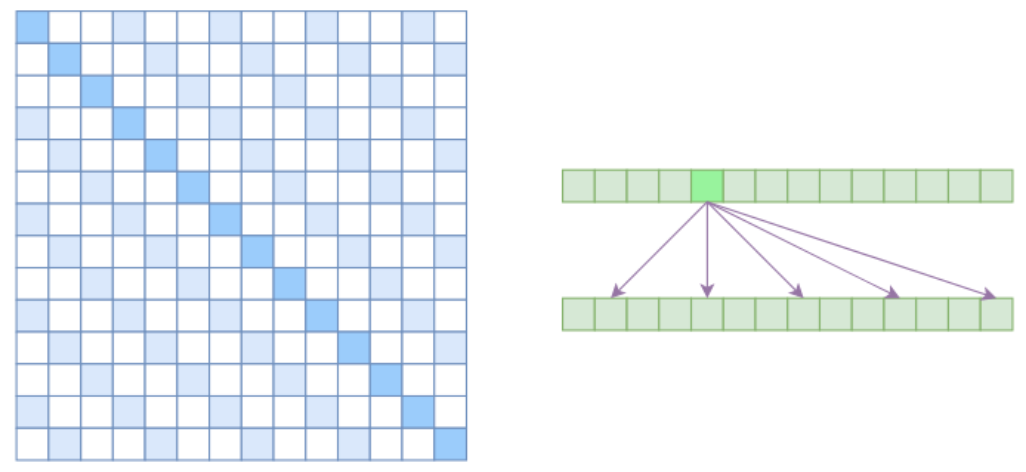### Local Self Attention

Local Self Attention中文可称之为“局部自注意力”。其实自注意力机制在CV领域统称为“Non Local”，而显然Local Self Attention则要放弃全局关联，重新引入局部关联。具体来说也很简单，就是约束每个元素只与前后k个元素以及自身有关联，如下图所示：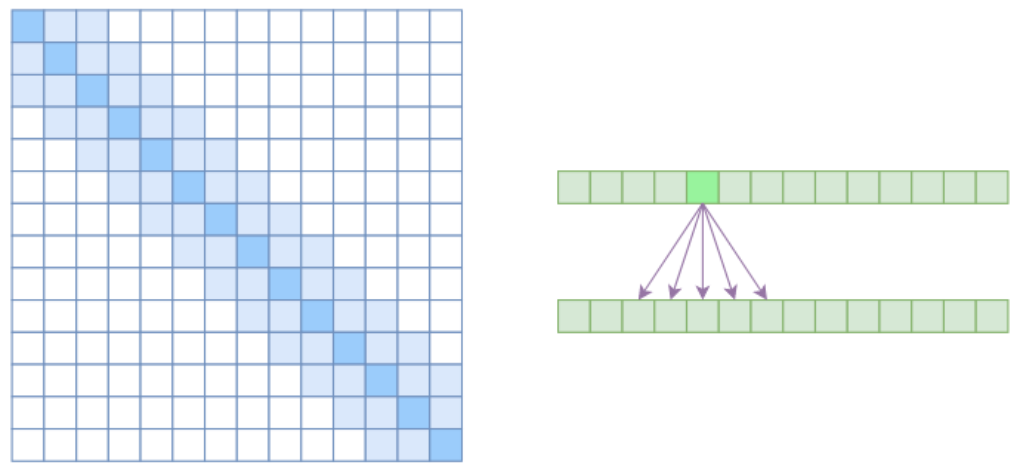### Sparse Self Attention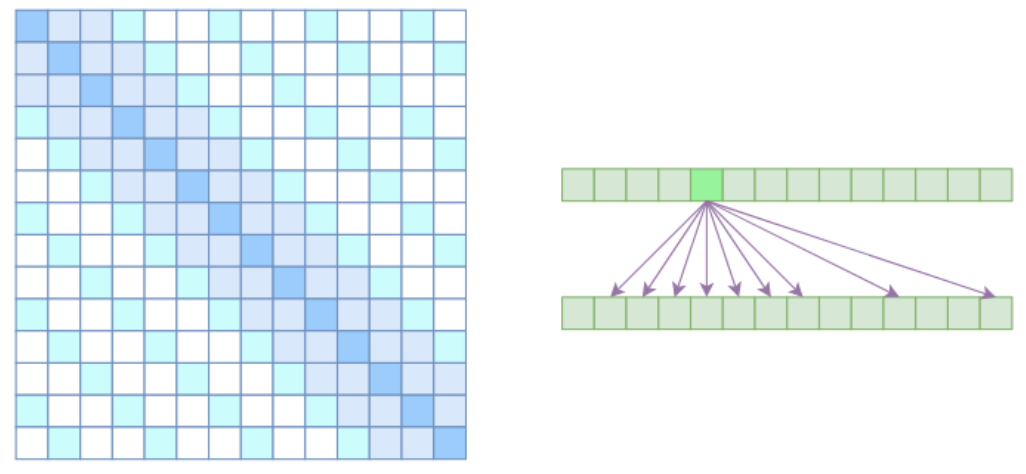## 线性注意力

$\text { Attention }(\boldsymbol{Q}, \boldsymbol{K}, \boldsymbol{V})_{i}=\frac{\sum_{j=1}^{n} e^{\boldsymbol{q}_{i}^{\top} \boldsymbol{k}_{j} \boldsymbol{v}_{j}}}{\sum_{j=1}^{n} e^{\boldsymbol{q}_{i}^{\top} \boldsymbol{k}_{j}}}$

$\text { Attention }(\boldsymbol{Q}, \boldsymbol{K}, \boldsymbol{V})_{i}=\frac{\sum_{j=1}^{n} \operatorname{sim}\left(\boldsymbol{q}_{i}, \boldsymbol{k}_{j}\right) \boldsymbol{v}_{j}}{\sum_{j=1}^{n} \operatorname{sim}\left(\boldsymbol{q}_{i}, \boldsymbol{k}_{j}\right)}$

## 基于距离自注意力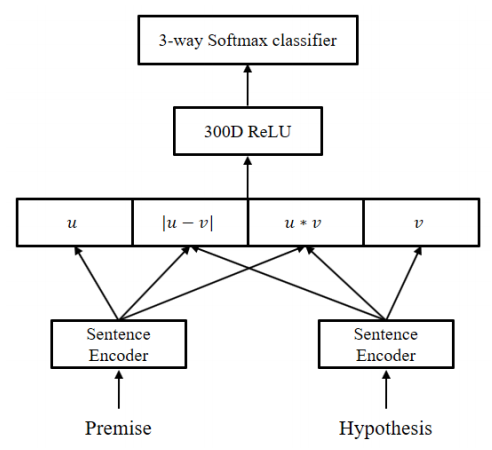sentence encoder 基于 self-attention 对句子进行编码 (如下), 可以看到中间那一部分像极了 Transformer 的 encoder. 不同点在于, mutl-head attention 带上了 mask, 后一层的 add 变成了 gate：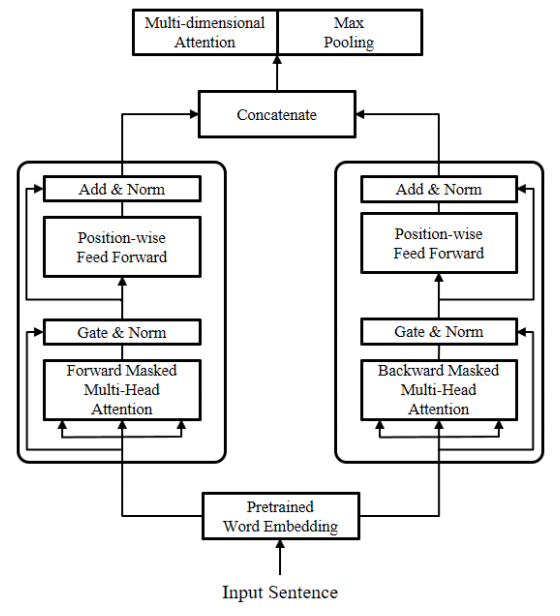$\begin{array}{l} \operatorname{Masked}(Q, K, V) \\ \quad=\operatorname{softmax}\left(\frac{Q K^{T}}{\sqrt{d_{k}}}+M_{d i r}+\alpha M_{d i s}\right) V \end{array}$

Distance mask 中每个元素代表句中两个单词间绝对距离的负. 由于$e^{-inf}=0$，因此距离越远, 负值越大, 单词间的依赖程度越低：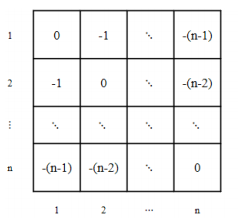Masked multi-head attention 之后是一个 Fusion gate, 控制 attention 输出和 word embedding 的比例：$\operatorname{Gate}(S, H)=F \odot S^{F}+(1-F) \odot H^{F}$

• distance mask 强化了单词对邻近单词的依赖, 但真正具有强依赖关系的单词, 在远距离的情况下也能保持依赖. 换言之, 在保证局部依赖的同时, 又不失全局依赖.
• Fusion gate 具有调节输出的作用, 关键词将更多地从 attention 输出, 非关键词更多地走 shortcut connection, 保持 word embedding.
• Multi-dimensional attention 与 max pooling 的行为很相似, 都更关注关键词.

## 全局和局部注意力

### Global Attention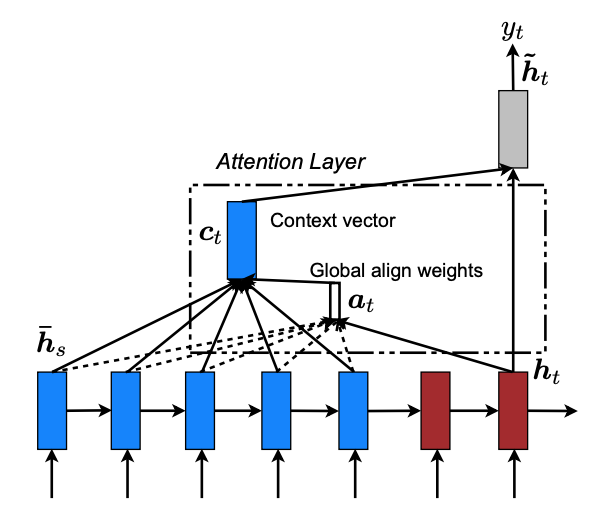Global Attention考虑Encoder顶层所有的隐藏状态，此时，对齐向量a_t的长度与输入的长度相同，也就是：a_{t}(s)=\operatorname{align}\left(h_{t}, \bar{h}_{s}\right)=\frac{\exp \left(\operatorname{score}\left(h_{t}, \bar{h}_{\mathrm{s}}\right)\right)}{\sum_{s^{\prime}} \exp \left(\operatorname{score}\left(h_{t}, \bar{h}_{s}\right)\right)}。

score其实就是计算当前隐层输出与源隐层输出的相似度比对，作者给出了score的三种content-based function：

$\operatorname{score}\left(\boldsymbol{h}_{t}, \overline{\boldsymbol{h}}_{s}\right)=\left\{\begin{array}{ll} \boldsymbol{h}_{t}^{\top} \overline{\boldsymbol{h}}_{s} & \text { dot } \\ \boldsymbol{h}_{t}^{\top} \boldsymbol{W}_{\boldsymbol{a}} \overline{\boldsymbol{h}}_{s} & \text { general } \\ \boldsymbol{v}_{a}^{\top} \tanh \left(\boldsymbol{W}_{\boldsymbol{a}}\left[\boldsymbol{h}_{t} ; \overline{\boldsymbol{h}}_{s}\right]\right) & \text { concat } \end{array}\right.$

### Local Attention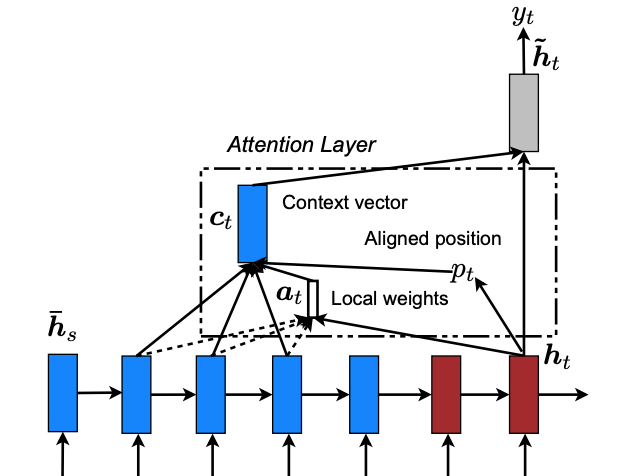Local Attention是这篇论文的重点部分，首先，Global Attention是有缺陷的，当输入的句子特别长的时候（比如输入是篇章级别的文档），Global Attention的计算量将会变得很大，因为我们要求源句子中所有的词都参与每一时刻的计算，所以作者才提出了Local Attention，即只注意源句子的一个小子集，而不是所有单词，这可以看作是一种软对齐和硬对齐的折中办法，不像软对齐那样注意所有的输入而导致计算量过大，也不像硬对齐那样只选择一个输入而导致过程不可微，需要加入复杂的技巧（variance reduction、reinforcement learning）来训练模型。因此Local Attention既可微，能训练，同时计算量小。

• Monotonic alignment：很简单，作者假设输入和输出在很大程度是一一对应的，直接设 $p_t=t$ 就行了，也就是假设输入和输出是单调对齐（Monotonic）的。有了窗口之后，后续步骤就和Global Attention相同了。
• Predictive alignment：不像上面那样假设输入输入单调对齐，而是预测对齐中心$p_{t}=S \cdot \operatorname{sigmoid}\left(\boldsymbol{v}_{p}^{\top} \tanh \left(\boldsymbol{W}_{\boldsymbol{p}} \boldsymbol{h}_{t}\right)\right)$，其中$W_p$、$v_p$为参数，S为源句子的长度，这样就有$p_{t} \in[0, S]$。另外，这里还有一个trick，作者对对齐向量的计算做了修改，作者在$p_t$周围引入了一个服从$N\left(p_{t}, D / 2\right)$的高斯分布来对齐权重，从直觉上考虑，距离目标位置越近的词理当起到更到的作用，因此对齐向量为\boldsymbol{a}_{t}(s)=\operatorname{align}\left(\boldsymbol{h}_{t}, \overline{\boldsymbol{h}}_{s}\right) \exp \left(-\frac{\left(s-p_{t}\right)^{2}}{2 \sigma^{2}}\right)，其中$\sigma$也是超参数，作者凭经验选择$\sigma=\frac{D}{2}$。同时也可以看到，在没有引入高斯分布之前，位置$p_t$并没有直接与网络相连，虽然计算$p_t$的过程可以微分，但是作为窗口中心这个操作是不可微的，因此也需要某种额外的方式（用$p_t$来导出$a_t$）将其与网络关联起来，使得参数可以通过backprop训练。
• 可以想下，没有Gassian的引入，是学习不了计算$p_t$中的$W_p$和$v_p$的，因为它不是直接通过某个函数和网络联系起来，而是计算出$p_t$的值，然后把它当做窗口的中心。虽然计算$p_t$的操作可以微分，但是当做窗口中心这个操作是没办法微分的，所以没办法BP。在Hard Attention中，直接取score最大的作为Attention，同理，这个取max的操作无法微分，所以Hard Attention需要使用其他technique来进行优化BP。

## 基于记忆的注意力

• $e_{i}=a\left(q, k_{i}\right)$(address memory)
• $\alpha_{\mathrm{i}}=\frac{\exp \left(\mathrm{e}_{\mathrm{i}}\right)}{\sum_{\mathrm{i}} \exp \left(\mathrm{e}_{\mathrm{i}}\right)}$(normalize)
• $c=\sum_{i} \alpha_{i} v_{i}$(read contents)

### Reusability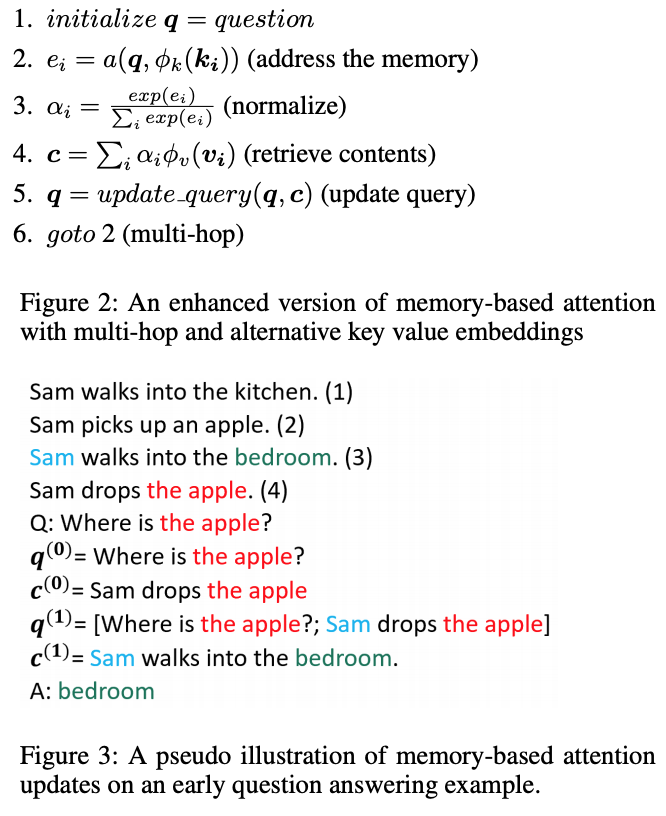## 基于强化学习的注意力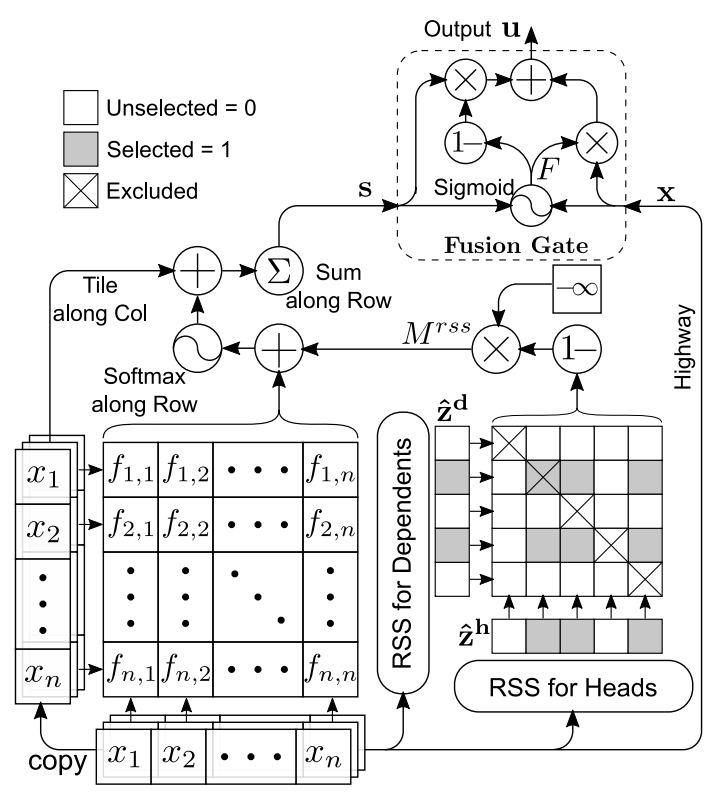Hard attention的目标是从输入序列中选择关键的words，这些关键的words能够提供足够的信息来完成下游任务，这样就可以排除掉许多boring words，从而减少模型的训练时间。

$g\left(f\left(x ; \theta_{f}\right)_{i} ; \theta_{g}\right)=\operatorname{sigmoid}\left(w^{T} \sigma\left(W^{(R)} f\left(x ; \theta_{f}\right)_{i}+b^{(R)}\right)+b\right)$

### ReSA

$\hat{z}^{h}=\left[\hat{z}_{1}^{h}, \ldots, \hat{z}_{n}^{h}\right] \sim \operatorname{RSS}\left(x ; \theta_{r h}\right)$

$\hat{z}^{d}=\left[\hat{z}_{1}^{d}, \ldots, \hat{z}_{n}^{d}\right] \sim \operatorname{RSS}\left(x ; \theta_{r d}\right)$

$M_{i j}^{r s s}=\left\{\begin{array}{ll} 0, & \hat{z}_{i}^{d}=\hat{z}_{j}^{h}=1 \& i \neq j \\ -\infty, & \text { otherwise } \end{array}\right.$

$f^{r s s}\left(x_{i}, x_{j}\right)=c \cdot \tanh \left(\left[W^{(1)} x_{i}+W^{(2)} x_{j}+b^{(1)}\right] / c\right)+M_{i j}^{r s s}$

$f^{r s s}\left(x_{i}, x_{j}\right)$即score function，然后使用softmax函数计算概率：

$P^{j}=\operatorname{softmax}\left(\left[f^{r s s}\left(x_{i}, x_{j}\right)\right]_{i=1}^{n}\right), \text { for } j=1, \ldots, n$

$x_j$的上下文注意力特性通过以下方式计算：

$s_{j}=\sum_{i=1}^{n} P_{i}^{j} \odot x_{i}, \text { for } j=1, \ldots, n$

$F=\operatorname{sigmoid}\left(W^{(f)}[\boldsymbol{x} ; s]+b^{(f)}\right)$

$\boldsymbol{u}=F \odot \boldsymbol{x}+(1-F) \odot \boldsymbol{s}$

## 多源注意力机制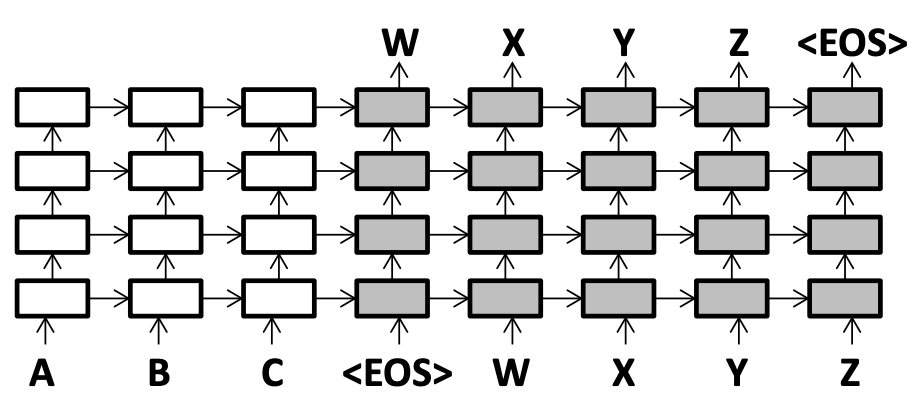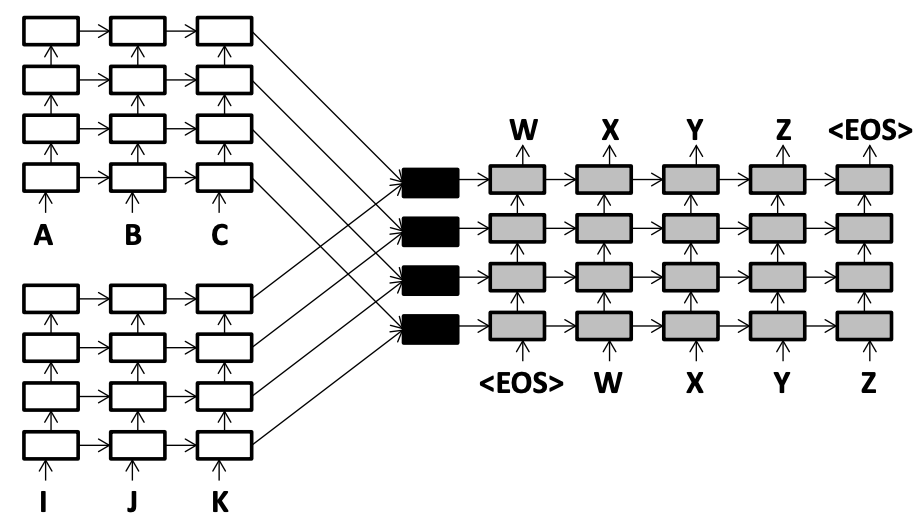### 状态融合方法

#### Child-Sum Method

• $i=\operatorname{sigmoid}\left(W_{1}^{i} h_{1}+W_{2}^{i} h_{2}\right)$代表输入门，是1000维的向量
• $f=\operatorname{sigmoid}\left(W_{i}^{f} h_{i}\right)$代表遗忘门，是1000维的向量
• $o=\operatorname{sigmoid}\left(W_{1}^{o} h_{1}+W_{2}^{o} h_{2}\right)$代表输出门，是1000维的向量
• $u=\tanh \left(W_{1}^{u} h_{1}+W_{2}^{u} h_{2}\right)$是1000维的向量
• $c=i_{f} \odot u_{f}+f_{1} \odot c_{1}+f_{2} \odot c_{2}$
• $h=o_{f} \odot \tanh \left(c_{f}\right) 上述公式中新增了8个矩阵，每个矩阵的尺寸都是1000*1000。 ### 多源注意力机制 本文中作为对比试验使用的单源注意力模型采用的是local-p attention model。decoder的隐藏状态可以回顾encoder的所有隐藏状态，从而学得一个更好的隐藏状态。local-p attention model采用如下方式操作：$p_{t}=S \cdot \operatorname{sigmoid}\left(v_{p}^{T} \tanh \left(W_{p} h_{t}\right)\right)$，S是源语句的长度。pt计算完之后用一个尺寸为(2D+1)的窗口在以pt（D＝10）为中心的源编码器的顶层中查看。对每一个窗口内的隐层状态，都计算一个0-1之间的对齐分数（权重）at(s)。计算方法如下： •$a_{t}(s)=\operatorname{align}\left(h_{t}, h_{s}\right) \exp \left(\frac{-\left(s-p_{t}\right)^{2}}{2 \sigma^{2}}\right)$，s是隐藏状态的源索引 •$\operatorname{align}\left(h_{t}, h_{s}\right)=\frac{\exp \left(\operatorname{score}\left(h_{t}, h_{s}\right)\right)}{\sum_{s^{\prime}} \exp \left(\operatorname{score}\left(h_{t}, h_{s^{\prime}}\right)\right)}$•$\operatorname{score}\left(h_{t}, h_{s}\right)=h_{t}^{T} W_{a} h_{s}$当所有对齐参数都被计算出来之后，ct是通过求所有源隐藏状态乘以它们的对齐权重的加权总和来创建的。最终送入softmax层的隐层状态为$\tilde{h}_{t}=\tanh \left(W_{c}\left[h_{t} ; c_{t}\right]\right)$。 论文中修改权重模型，同时查看两个源的encoder。为每一个encoder创建一个上下文向量ct1 和ct2（在单源模型中只有ct），即：$\tilde{h_{t}}=\tanh \left(W_{c}\left[h_{t} ; c_{t}^{1} ; c_{t}^{2}\right]\right)$。因此在修改之后的注意力机制模型中有两个pt变量。还有两套不同的对齐参数。还有两个ct值表示为上述的c1t和c2t。 在对话方向上，也有一篇论文《Two are Better than One: An Ensemble of Retrieval- and Generation-Based Dialog Systems》借鉴了该思想，具体思想可参考知乎文章：https://zhuanlan.zhihu.com/p/31277046 ## 注意力之上的注意力 论文《Attention-over-Attention Neural Networks for Reading Comprehension》使用一种新颖的模式解决了完形填空式阅读理解任务。其具体过程如下： • 获取document hdoc和query hquery的文本嵌入 • 把document D和query Q中的每个单词都转换为一个one-hot表示 • 用一个共享嵌入矩阵We把它们转换为连续表示$e(x)=W_{e} \cdot x, \text { where } x \in \mathcal{D}, \mathcal{Q}$• 使用两个双向rnn来获取query和document的文本表示，其中每一个词的表达都是通过前向和后向隐藏层的拼接，使用GRU来作为使用的循环单元 •$\overrightarrow{h_{s}(x)}=\overrightarrow{G R U}(e(x))$•$\overleftarrow{h_{s}(x)}=\overleftarrow{G R U}(e(x))$•$h_{s}(x)=\left[\overrightarrow{h_{s}(x)} ; \overleftarrow{h_{s}(x)}\right]$•$h_{d o c} \in \mathbb{R}^{|\mathcal{D}| * 2 d}$•$h_{q u e r y} \in \mathbb{R}|\mathcal{Q}| * 2 d$• 计算pair-wise匹配矩阵：$M(i, j)=h_{d o c}(i)^{T} \cdot h_{q u e r y}(j)$表示document的第i个词和query的第j个词的匹配分数，依据这个分数可以构造矩阵$M \in \mathbb{R}^{|\mathcal{D}| * \mid \mathcal{Q}}$• 计算query-to-document attention：应用一个column-wise的softmax函数来得到了每一列的概率分布，每一列都是考虑一个query词的document的attention分布。我们把时间t（query）处的document attention分布称作$\alpha(t) \in \mathbb{R}^{|\mathcal{D}|}$，其中$\alpha(t)=\operatorname{softmax}(M(1, t), \ldots, M(|\mathcal{D}|, t))$，$\alpha=[\alpha(1), \alpha(2), \ldots, \alpha(|\mathcal{Q}|)]$• 计算document-to-query attention：用一个row-wise softmax函数来对pair-wise匹配矩阵M来得到query级别的attention，对于一个在时间t的document词，我们计算query中每个词对他的重要性分布，来表明query中哪个词对这个document词更加重要。把时间t（document）处的query attention分布称作$\beta(t) \in \mathbb{R}^{|\mathcal{Q}|}$，其中$\beta(t)=\operatorname{softmax}(M(t, 1), \ldots, M(t,|\mathcal{Q}|))$• 计算document和query的交互信息 • 平均所有的β（t）来得到一个平均的query上的attention β：$\beta=\frac{1}{n} \sum_{t=1}^{|\mathcal{D}|} \beta(t)$• 计算α和β之间的点积：$s=\alpha^{T} \beta$，$s \in \mathbb{R}^{|\mathcal{D}|}$这个操作就是看着query的词汇t的时候，计算所有document单体attention的加权和。这样，每一个query词汇的贡献度就能直接学习到。 • 预测结果：用sum attention mechanism 方法来聚合结果。注意最后的输出应该被reflected 到词汇空间V，而不是document级别的attention |D|，这会在性能上引起很大差异：$P(w \mid \mathcal{D}, \mathcal{Q})=\sum_{i \in I(w, \mathcal{D})} s_{i}, w \in V$• 其中I（w,D）表示词汇w出现在document中的位置的集合。 • 作为训练目标，我们最大化正确答案的log函数：$\mathcal{L}=\sum_{i} \log (p(x)), x \in \mathcal{A}$整体计算过程如下图所示：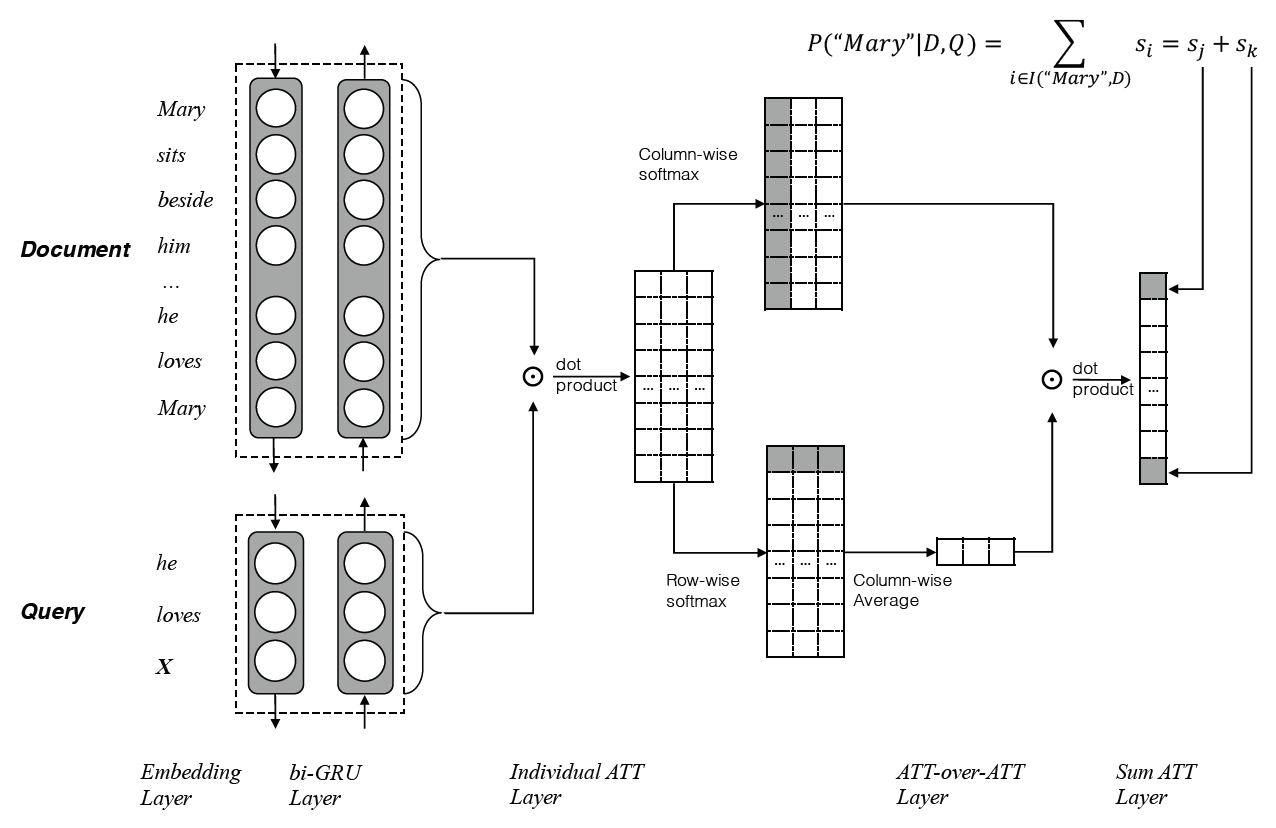## 多跳注意力机制 论文《Convolutional Sequence to Sequence Learning》使用encoder-decoder + attention模块的大框架来处理机器翻译的任务：encoder 和 decoder采用了相同的卷积结构，其中的非线性部分采用的是门控结构 gated linear units（GLM）；attention 部分采用的是多跳注意 multi-hop attention，也即在 decoder 的每一个卷积层都会进行 attention 操作，并将结果输入到下一层。其具体过程如下： • Position Embeddings • 词向量：$w=\left(w_{1}, \ldots, w_{n}\right)$• 位置向量：$p=\left(p_{1}, \ldots, p_{n}\right)$• 最终表示向量：$e=\left(w_{1}+p_{1}, \ldots, w_{n}+p_{n}\right)$• Convolutional Block Structure • encoder 和 decoder 都是由 l 层卷积层构成，encoder输出为$z^l $，decoder输出为$h^l$，由于卷积网络是层级结构，通过层级叠加能够得到远距离的两个词之间的关系信息 • 卷积计算：卷积核的大小为$W \in \mathbb{R}^{2 d \times k d}$，其中d为词向量长度，k为卷积窗口大小，每次卷积生成两列d维向量$Y=[A B] \in \mathbb{R}^{2 d}$• 非线性计算：非线性部分采用的是门控结构 gated linear units（GLM），即$v([A, B])=A \otimes \delta(B)$，其中$\delta(B)$是门控函数，控制着网络中的信息流，即哪些能够传递到下一个神经元中。 • 残差连接：$h_{i}^{l}=v\left(W^{l}\left[h_{i-k / 2}^{l-1}, \ldots, h_{i+k / 2}^{l-1}\right]+b^{l}\right)+h_{i}^{l-1}$• Decoder输出：$p\left(y_{i+1} \mid y_{1}, \ldots, y_{i}, x\right)=\operatorname{softmax}\left(W_{o} h_{i}^{L}+b_{o}\right)$• Multi-step Attention •$d_{i}^{l}=W_{d}^{l} h_{i}^{l}+b_{d}^{l}+g_{i}$•$a_{i j}^{l}=\frac{\exp \left(d_{i}^{l} \cdot z_{j}^{u}\right)}{\sum_{t=1}^{m} \exp \left(d_{i}^{l} \cdot z_{t}^{u}\right)}$为权重信息，采用了向量点积的方式再进行softmax操作，这里向量点积可以通过矩阵计算，实现并行计算 •$c_{i}^{l}=\sum_{j=1}^{m} a_{i j}^{l}\left(z_{j}^{u}+e_{j}\right)$•$h_i = c_i + h_i每一个卷积层都会进行 attention 的操作，得到的结果输入到下一层卷积层，这就是多跳注意机制multi-hop attention。这样做的好处是使得模型在得到下一个主意时，能够考虑到之前的已经注意过的词。 模型的整体结构如下图：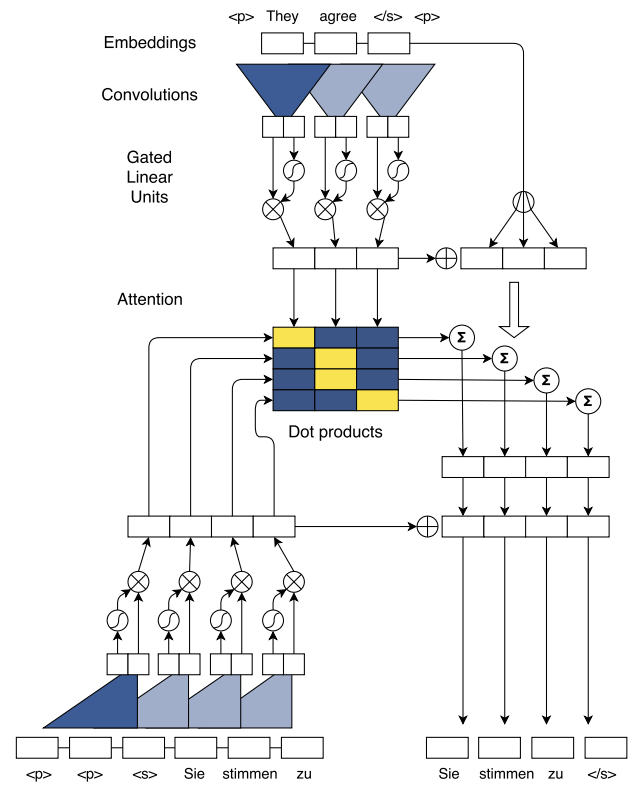## 多维度注意力 ### DiSAN 论文《DiSAN: Directional Self-Attention Network for RNN/CNN-Free Language Understanding》创新性地提出了 2 种 attention mechanism: multi-dimensional attention 和 directional attention。 前者用矩阵代替向量来表示 attention weights, 将加权求和细化到状态向量的每一维; 后者通过 Mask 矩阵将方向编码进 attention, 解决了时序丢失问题. 基于两种 attention提出了 Directional Self-Attention Network, DiSAN 模型, 在不依赖 RNN/CNN 的情况下, 在多项任务 NLP 任务上取得了 SOTA 的成绩. #### Multi-Dimensional Attention 普通 attention 的 alignment score 是一个标量即f\left(x_{i}, q\right)=w^{T} \sigma\left(W^{(1)} x_{i}+W^{(2)} q\right)$，之后、$a=\left[f\left(x_{i}, q\right)\right]_{i=1}^{n}$、$p(z \mid \boldsymbol{x}, q)=\operatorname{softmax}(a)$得到的 attention weight p_i也是一个标量, 最后计算输出状态$s=\sum_{i=1}^{n} p(z=i \mid \boldsymbol{x}, q) x_{i}=\mathbb{E}_{i \sim p(z \mid \boldsymbol{x}, q)}\left(x_{i}\right)$，$s \in \mathbb{R}^{d_{e}}$是句子表示。 Multi-dimensional attention 的依据是x_k的每一位数字都是一个特征 (比如 king 的词向量, 某一位数字表征性别, 某一位数字表征尊贵程度), 可以拥有自己的 attention weight, 文中称为 feature-wise attention. 具体做法就是将上面 f 函数中的权值向量替换为权值矩阵：$f\left(x_{i}, q\right)=W^{T} \sigma\left(W^{(1)} x_{i}+W^{(2)} q+b^{(1)}\right)+b$如此得到的$z_i$将是与$x_i$具有相同 embedding dimension 的向量, 该向量的每一位数字表征$x_i$与 q 相应位置上的对齐程度。 后续的计算过程与上总体无异, 只是 z 成了矩阵, softmax 应用于 z 的第二维，如下图(b)：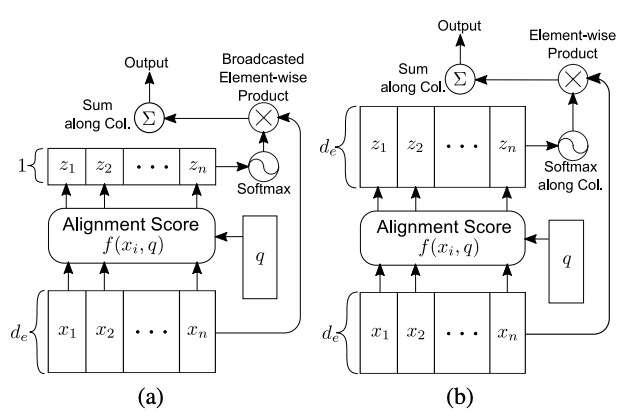Multi-dimensional attention 对于同一个 token embedding 的不同 feature 计算不同的 attention weight, 充分利用了上下文与 token 不同特征之间的对齐程度. 还是以 king 为例, 当上下文强调性别属性时, 性别特征将得到更大的 attention weight, 而尊贵程度不那么重要, 可能就只分配很小的 attention weight。 对于一词多义的情况, multi-dimensional attention 还具有一定的消除歧义作用。 在 multi-dimensional attention 的基础上, 文章得到了 2 个 self-attention 变种, 两者的差别在于 query: • token2token: 计算序列中两个位置的依赖程度 (对于 self-attention, 依赖的说法比对齐更合适), 此时只需将外部 query q 替换为序列中另一个位置的状态即可(由于需要计算位置两两之间的对齐程度, token2token 的输出是一个矩阵)：$f\left(x_{i}, x_{j}\right)=W^{T} \sigma\left(W^{(1)} x_{i}+W^{(2)} x_{j}+b^{(1)}\right)+b$• source2token: 计算序列某位置状态与整个序列的对齐程度, 文章简单地将 query 剔除了(source2token 的 输出是向量)：$f\left(x_{i}\right)=W^{T} \sigma\left(W^{(1)} x_{i}+b^{(1)}\right)+b$#### Directional Self-Attention Directional self-attention (DiSA) 由一个全连阶层 FC, 一个 masked multi-dimensional token2token self-attention block, 一个 fusion gate 组成： • FC 将输入序列转换成隐藏状态的序列 • multi-dimensional token2token self-attention 学习各隐藏状态之间的关系, 并使用 Mask 将方向编码进结果 • 通过 mask 矩阵, 实现了非对称依赖, 即h_j对h_i有依赖, 反之却没有， 从而实现了方向性 • Mask 是一个非零即负无穷的方阵, 因为负无穷的指数为0，从而能抑制依赖关系 • 上式可修改为$\begin{array}{l}
f\left(h_{i}, h_{j}\right)= \\
c \cdot \tanh \left(\left[W^{(1)} h_{i}+W^{(2)} h_{j}+b^{(1)}\right] / c\right)+M_{i j} \mathbf{1}
\end{array}$• 文中使用了 forward mask 与 backward mask， 前者的作用是使得只存在后面状态对前面状态的依赖;，后者则恰好相反：• fusion gate 是一个门控单元, 控制 DiSA 中 FC 的输出与 token2token 输出的比例 • Fusion gate 是 dimension-wise 的, 即全面控制输出结果向量的每一位数字 •$F=\operatorname{sigmoid}\left(W^{(f 1)} s+W^{(f 2)} \boldsymbol{h}+b^{(f)}\right)$•$\boldsymbol{u}=F \odot \boldsymbol{h}+(1-F) \odot \boldsymbol{s}$最终, DiSAN 由两个 DiSA blocks 和一个 multi-dimensional source2token self-attention layer 组成. 通过 DiSA 分别对前向与反向 上下文依赖 context dependency 进行建模, 为每一个 token 生成一个上下文感知 context-aware 的表示; 然后用 source2token 计算整个序列的向量表示：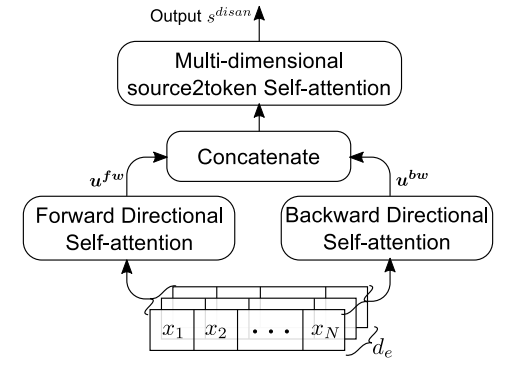## 基于拷贝机制的注意力 ### PtrNet 论文《Pointer Networks》提出了一种新的指针网络，它主要解决的是Seq2Seq Decoder端输出的词汇表大小不可变的问题。换句话说，传统的Seq2Seq无法解决输出序列的词汇表会随着输入序列长度的改变而改变的那些问题，而某些问题的输出可能会严重依赖于输入。 我们已经知道，Seq2Seq的出现很好地解开了以往的RNN模型要求输入与输出长度相等的约束，而其后的Attention机制（content-based）又很好地解决了长输入序列表示不充分的问题。尽管如此，这些模型仍旧要求输出的词汇表需要事先指定大小，因为在softmax层中，词汇表的长度会直接影响到模型的训练和运行速度，因此人们往往会丢弃生词，保留高频词。之前也有人采用过一些简单的trick解决生词问题，但都不如Copy机制那么有效。下图是PtrNet结构：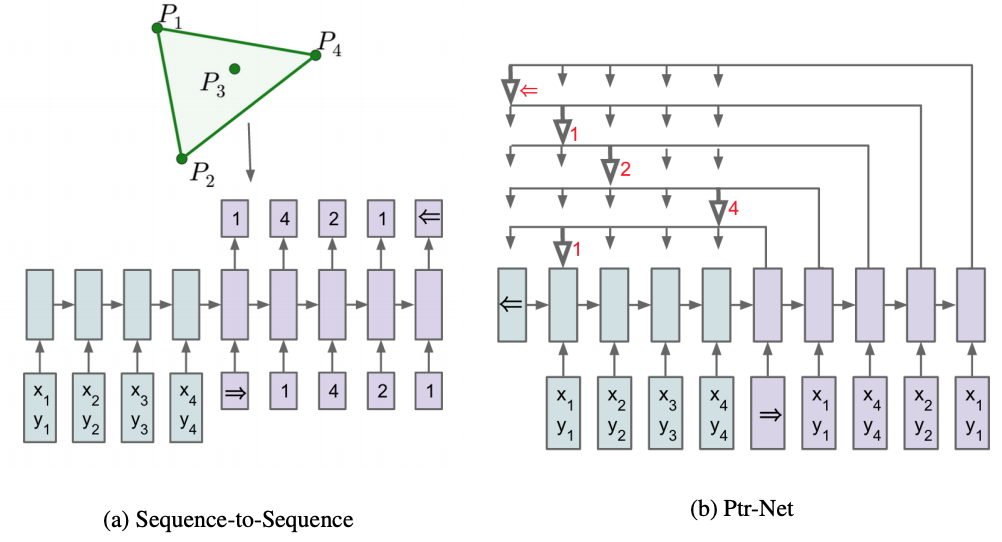不难想象，Seq2Seq需要词汇表固定的原因是在预测输出时，模型采用了Softmax distribution来计算生成词汇表中每一个词的似然概率，而对于这里的几何问题来说，输出集合是与输入相关联的，为了解决这个问题，作者将Attention机制改为了如下形式： •$u_{j}^{i}=v^{T} \tanh \left(W_{1} e_{j}+W_{2} d_{i}\right) \quad j \in(1, \ldots, n)$•$p\left(C_{i} \mid C_{1}, \ldots, C_{i-1}, \mathcal{P}\right)=\operatorname{softmax}\left(u^{i}\right)$第一个式子和Attention一样，即计算当前输出与对应输入之间的相关性分数，然后对其进行softmax归一化得到权重，在这里，PrtNets直接将权重最大的输入（也就是所谓指针指向的输入）作为输出，也就是直接copy注意力得分最高的输入作为输出。实际上，传统的带有注意力机制的Seq2Seq模型输出的是针对输出词汇表的一个概率分布，而Pointer Networks输出的则是针对输入文本序列的概率分布。虽然简单，但十分有用，由于输出元素来自输入元素的特点，PtrNets特别适合用来直接复制输入序列中的某些元素给输出序列。这对于那些输出为输入的position的任务非常有效。 论文《Get To The Point: Summarization with Pointer-Generator Networks》融合了seq2seq模型和pointer network的pointer-generator network以及覆盖率机制(coverage mechanism)应用于摘要生成。模型主要框架如下：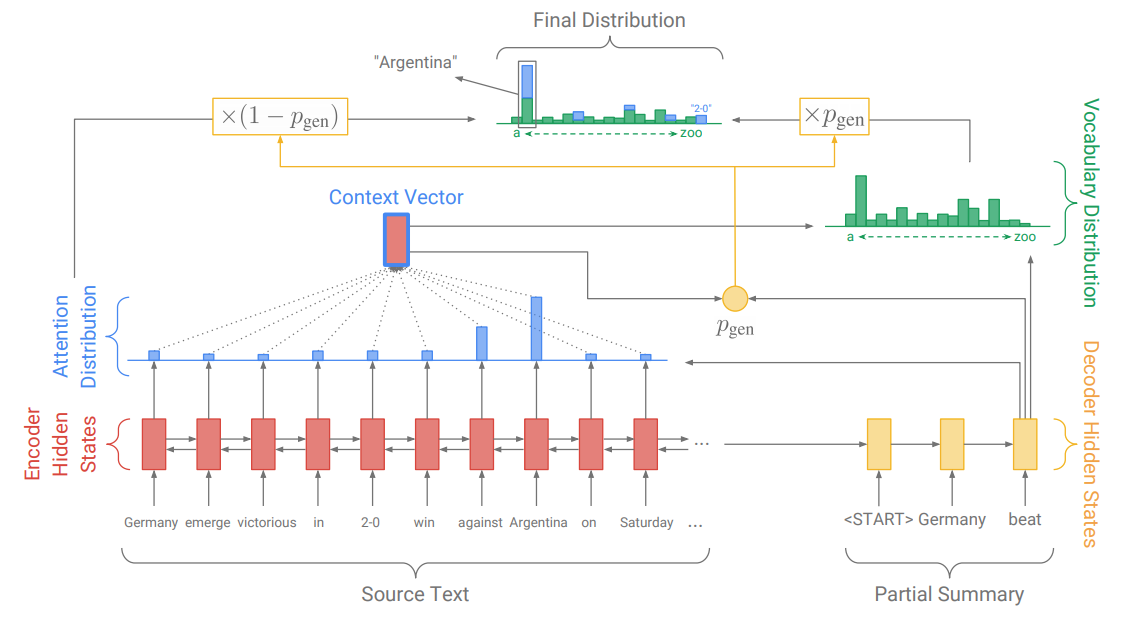我们知道，对于普通的seq2seq模型的attention计算公式为： •$e_{i}^{t}=v^{T} \tanh \left(W_{h} h_{i}+W_{s} s_{t}+b_{\mathrm{attn}}\right)$，$h_i$是输出的编码隐层状态，$s_t$是解码的隐层状态 •$a^{t}=\operatorname{softmax}\left(e^{t}\right)$•$h_{t}^{*}=\sum_{i} a_{i}^{t} h_{i}$对encoder输出的隐层做加权平均，获得原文的动态表示，称为语境向量 •$P_{\text {vocab }}=\operatorname{softmax}\left(V^{\prime}\left(V\left[s_{t}, h_{t}^{*}\right]+b\right)+b^{\prime}\right)$依靠decoder输出的隐层和语境向量，共同决定当前步预测在词表上的概率分布 •$P(w)=P_{\text {vocab }}(w)$•$\operatorname{loss}_{t}=-\log P\left(w_{t}^{*}\right)$Pointer-generator network是seq2seq模型和pointer network的混合模型，一方面通过seq2seq模型保持抽象生成的能力，另一方面通过pointer network直接从原文中取词，提高摘要的准确度和缓解OOV问题。在预测的每一步，通过动态计算一个生成概率$p_{gen}$，把二者软性地结合起来：$p_{\text {gen }}=\sigma\left(w_{h^{}}^{T} h_{t}^{}+w_{s}^{T} s_{t}+w_{x}^{T} x_{t}+b_{\mathrm{ptr}}\right)$文章对pointer network的处理比较巧妙，直接把seq2seq模型计算的attention分布作为pointer network的输出，通过参数复用，大大降低了模型的复杂度，最终的预测为：$P(w)=p_{\text {gen }} P_{\text {vocab }}(w)+\left(1-p_{\text {gen }}\right) \sum_{i: w_{i}=w} a_{i}^{t}$论文额外还增加了一个coverage向量$c^{t}=\sum_{t^{\prime}=0}^{t-1} a^{t^{\prime}}$计算过去所有预测步计算的attention分布的累加和，记录着模型已经关注过原文的哪些词,并且让这个coverage向量影响当前步的attention计算：$e_{i}^{t}=v^{T} \tanh \left(W_{h} h_{i}+W_{s} s_{t}+w_{c} c_{i}^{t}+b_{\mathrm{attn}}\right)$。这样做的目的在于，在模型进行当前步attention计算的时候，告诉它之前它已经关注过的词，希望避免出现连续attention到某几个词上的情形。同时，coverage模型还添加一个额外的coverage loss，来对重复的attention作惩罚：$\text { covloss }_{t}=\sum_{i} \min \left(a_{i}^{t}, c_{i}^{t}\right)$。值得注意的是这个loss只会对重复的attention产生惩罚，并不会强制要求模型关注原文中的每一个词。最终，模型的整体损失函数为：$\operatorname{loss}_{t}=-\log P\left(w_{t}^{*}\right)+\lambda \sum_{i} \min \left(a_{i}^{t}, c_{i}^{t}\right)$。不过文章在实验部分提到，如何移除了covloss，单纯依靠coverage向量去影响attention的计算并不能缓解重复问题，模型还是会重复地attention到某些词上。而加上covloss的模型训练上也比较trick，需要先用主函数训练好一个收敛的模型，然后再把covloss加上，做个finetune，不然的话效果还是不好。 ### CopyNet 论文《Incorporating Copying Mechanism in Sequence-to-Sequence Learning》提出了CopyNet，在 Seq2Seq + Attention 的基础上，引入了拷贝机制，模型结构如下图：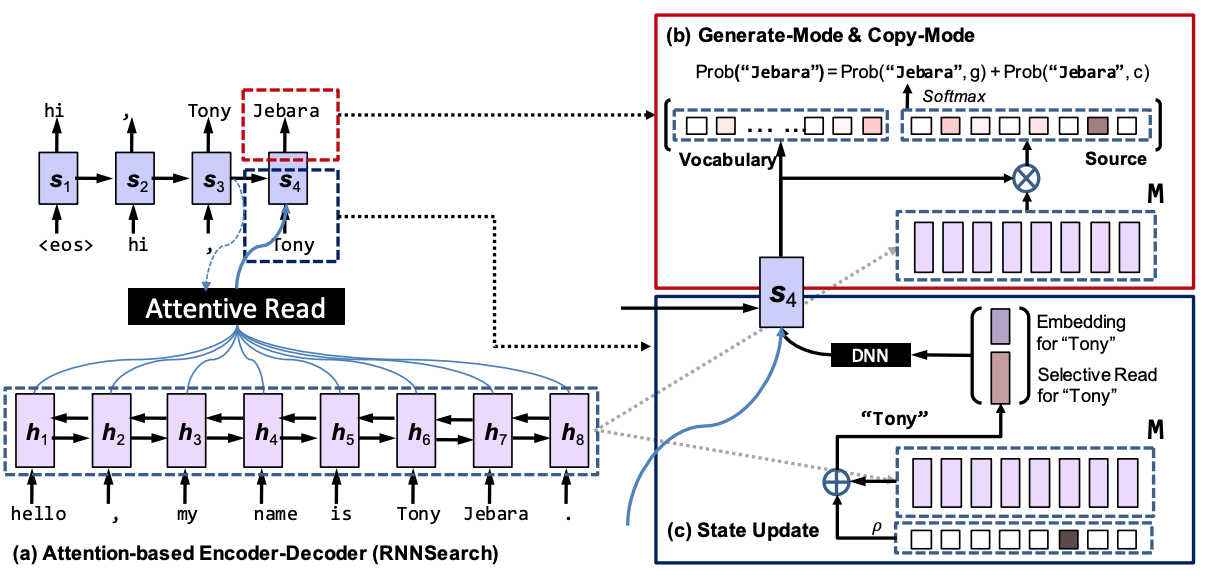一般的encoder－decoder结构和基于attention的encoder－decoder结构都严重依赖于input的表征，很难处理output中需要copy input中一些实体名字的问题。本文提出的COPYNET就可以兼顾表征和copy。还有个问题就是，copy这个操作是一个0-1操作，意思就是选择copy时是1，不选择copy是0，这种硬选择很难优化，本文还是用end－end的网络进行了训练。模型的具体计算过程如下： • encoder：bi-directional RNN 得到source sentence的定长hidden states。每个h_t 对应一个source word$x_t$，source sentence的表征就变成了$\{h_1, …, h_{T_s}\}$(文中用符号M表示) • decoder：把M输入到RNN中，预测target sequence。 • Prediction：从decoder hidden state s_t到预测输出y_t的过程，本来是一个线性映射就可以，现在要用generate-mode和copy-mode的混合概率预测：$p\left(y_{t} \mid s_{t}, y_{t-1}, c_{t}, M\right)=p\left(y_{t}, g \mid s_{t}, y_{t-1}, c_{t}, M\right)+p\left(y_{t}, c \mid s_{t}, y_{t-1}, c_{t}, M\right)$• Generate-Mode：对输出词表$\mathcal{V}=\left\{v_{1}, \ldots v_{N}\right\}$中的单词$v_i$（one-hot表示），未登录词UNK，输入词$\mathcal{X}$有： •$\psi_{g}\left(y_{t}=v_{i}\right)=\mathbf{v}_{i}^{\top} \mathbf{W}_{o} \mathbf{s}_{t}, \quad v_{i} \in \mathcal{V} \cup \mathrm{UNK}$，其中$\mathbf{W}_{o} \in \mathbb{R}^{(N+1) \times d_{s}}$•$p\left(y_{t}, \mathrm{~g} \mid \cdot\right)=\left\{\begin{array}{cc}
\frac{1}{Z} e^{\psi_{g}\left(y_{t}\right)}, & y_{t} \in \mathcal{V} \\
0, & y_{t} \in \mathcal{X} \cap \bar{V} \\
\frac{1}{Z} e^{\psi_{g}(\mathrm{UNK})} & y_{t} \notin \mathcal{V} \cup \mathcal{X}
\end{array}\right.$•$Z=\sum_{v \in \mathcal{V} \cup\{U N K\}} e^{\psi_{g}(v)}+\sum_{x \in X} e^{\psi_{c}(x)}$• Copy-Mode：论文模型不仅基于内容（the content），也基于句子的信息位置（location information），因此当计算得分函数时，用$\{h_1, …, h_{T_S}\}$来替代源句子$\{x_1, …, x_{T_S}\}$，因此对输入中出现的单词$\mathcal{X}$有： •$\psi_{c}\left(y_{t}=x_{j}\right)=\sigma\left(\mathbf{h}_{j}^{\top} \mathbf{W}_{c}\right) \mathbf{s}_{t}, \quad x_{j} \in \mathcal{X}$，其中$\mathbf{W}_{c} \in \mathbb{R}^{d_{h} \times d_{s}}$，$d_h$、$d_s$是$h_j$、$s_t$的维度 •$p\left(y_{t}, \mathrm{c} \mid \cdot\right)=\left\{\begin{array}{cc}
\frac{1}{Z} \sum_{j: x_{j}=y_{t}} e^{\psi_{c}\left(x_{j}\right)}, & y_{t} \in \mathcal{X} \\
0 & \text { otherwise }
\end{array}\right.$• State Update：对于seq2seq中的Attention，发生在更新$s_t$上，即$\mathbf{s}_{t}=f\left(y_{t-1}, \mathbf{s}_{t-1}, \mathbf{c}_{t}\right)$其中$c_t$是context vector，也就是attention模块，其计算公式如下：$\mathbf{c}_{t}=\sum_{\tau=1}^{T_{S}} \alpha_{t \tau} \mathbf{h}_{\tau}, \quad \alpha_{t \tau}=\frac{e^{\eta\left(\mathbf{s}_{t-1}, \mathbf{h}_{\tau}\right)}}{\sum_{\tau^{\prime}} e^{\eta\left(\mathbf{s}_{t-1}, \mathbf{h}_{\tau^{\prime}}\right)}}$，CopyNet的$y_{t-1}$在这里有些不同，这里被表示成了$\left[\mathbf{e}\left(y_{t-1}\right) ; \zeta\left(y_{t-1}\right)\right]^{\top}$，前一项是word embedding，多一项的后一项叫做selective read，是为了能连续拷贝较长的短语： •$\zeta\left(y_{t-1}\right)=\sum_{\tau=1}^{T_{S}} \rho_{t \tau} \mathbf{h}_{\tau}$表示M中和$y_t$对应的hidden states加权 •$\rho_{t \tau}=\left\{\begin{array}{cc}
\frac{1}{K} p\left(x_{\tau}, \mathbf{c} \mid \mathbf{s}_{t-1}, \mathbf{M}\right), & x_{\tau}=y_{t-1} \\
0 & \text { otherwise }